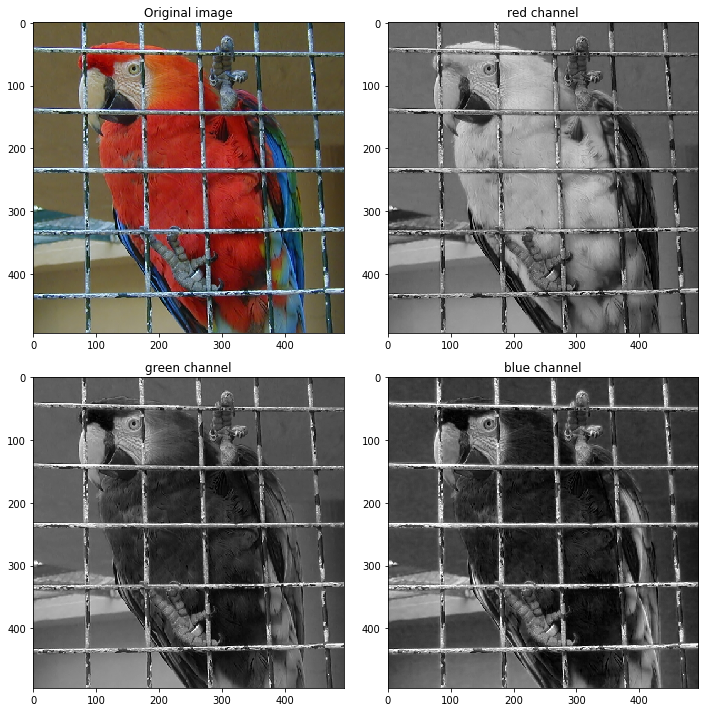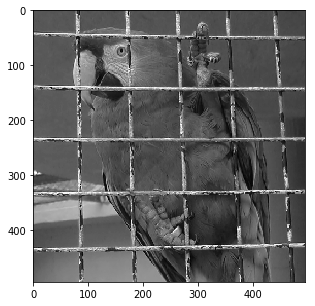# Introduction to image processing - Color in images

## Introduction¶

For the following exercices, you need Python 3 with some basic librairies (see below). All images necessary for the session are available here.

If you use your own Python 3 install, you should download the images, put them in a convenient directory and update the path in the next cell.

For some parts of the session (cells with commands written as todo_something...), you are supposed to code by yourself.

In :
path = '../im/'

In :
import numpy as np
import matplotlib.pyplot as plt
import matplotlib
%matplotlib inline
import scipy.signal as scs
from mpl_toolkits.mplot3d import Axes3D
from sklearn.cluster import KMeans


The following line will be used to import the solutions of the practical session. Do not use it for the moment.

In :
from TP_color import *


Load and display a color image. A color image is made of three channels : red, green and blue. A color image in $\mathbb{R}^{N\times M}$ is stored as a $N\times M\times 3$ matrix.

Be careful with the functions plt.imread() and plt.imshow() of matplotlib.

• plt.imread() reads png images as numpy arrays of floating points between 0 and 1, but it reads jpg or bmp images as numpy arrays of 8 bit integers.

• In this practical session, we assume floating point images between 0 and 1, so if you use jpg or bmp images, you should normalize them to $[0,1]$.

• If 'im' is an image encoded as a double numpy array, plt.imshow(im) will display all values above 1 in white and all values below 0 in black. If the image 'im' is encoded on 8 bits though, plt.imshow(im) will display 0 in black and 255 in white.</span>

In :
imrgb =plt.imread(path+"parrot.png")

# extract the three (red,green,blue) channels from imrgb
imred   = imrgb[:,:,0]
imgreen = imrgb[:,:,1]
imblue  = imrgb[:,:,2]

#image size
[nrow,ncol,nch]=imrgb.shape
fig, axes = plt.subplots(nrows=2, ncols=2, figsize=(10, 10))

#we display the images
axes[0, 0].imshow(imrgb)
axes[0,0].set_title('Original image')
axes[0, 1].imshow(imred, cmap="gray")
axes[0,1].set_title('red channel')
axes[1, 0].imshow(imgreen, cmap="gray")
axes[1,0].set_title('green channel')
axes[1, 1].imshow(imblue, cmap="gray")
axes[1,1].set_title('blue channel')
fig.tight_layout()It might be useful to convert the color image to gray level. This can be done by averaging the three channels, or by computing another well chosen linear combination of the coordinates R, G and B.

In :
imgray = np.sum(imrgb,2)/3
plt.figure(figsize=(5, 5))
plt.imshow(imgray,cmap='gray')

Out:
<matplotlib.image.AxesImage at 0x10c545d68>## Color spaces¶

### Opponent spaces¶

Color opponent spaces are characterized by a channel representing an achromatic signal, as well as two channels encoding color opponency. The two chromatic channels generally represent an approximate red-green opponency and yellow- blue opponency. $$O_1 = \frac 1 {\sqrt{2}} (R-G),\; O_2 = \frac 1 {\sqrt{6}} (R+G-2B),\; O_3 = \frac 1 {\sqrt{3}} (R+G+B)$$

• Display the O_1, O_2 and O_3 coordinates for different color images.
In :
O1 = (imred-imgreen)/np.sqrt(2)
O2 = (imred+imgreen-2*imblue)/np.sqrt(6)
O3 = (imred+imgreen+imblue)/np.sqrt(3)

fig, axes = plt.subplots(nrows=1, ncols=3, figsize=(15, 10))

axes.imshow(O1, cmap='gray')
axes.set_title('O1')
axes.imshow(O2, cmap='gray')
axes.set_title('O2')
axes.imshow(O3, cmap='gray')
axes.set_title('O3')
fig.tight_layout()